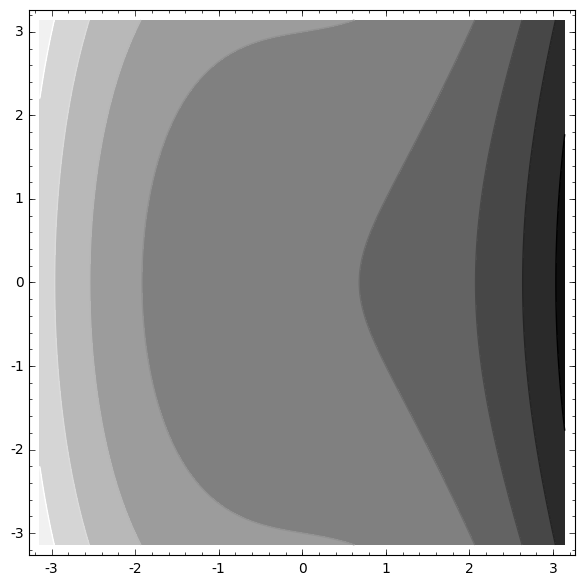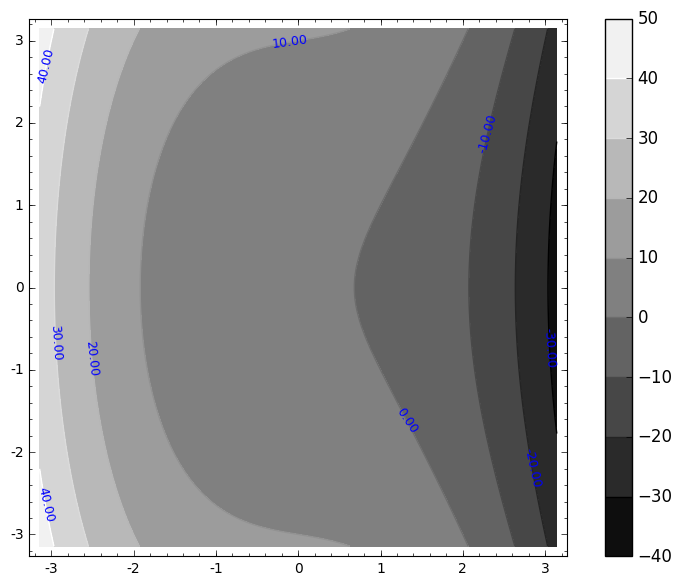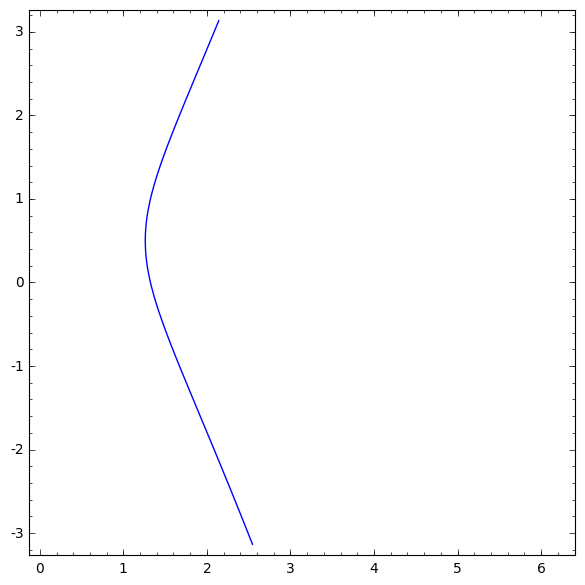A Jupyter Notebook from a SageMath tutorial

I gave an introduction to sage tutorial at the University of Warwick Computational Group seminar today, 2 November 2017. Below is a conversion of the sage/jupyter notebook I based the rest of the tutorial on. I said many things which are not included in the notebook, and during the seminar we added a few additional examples and took extra consideration to a few different calls. But for reference, the notebook is here.

The notebook itself (as a jupyter notebook) can be found and viewed on my github (link to jupyter notebook). When written, this notebook used a Sage 8.0.0.rc1 backend kernel and ran fine on the standard Sage 8.0 release , though I expect it to work fine with any recent official version of sage. The last cell requires an active notebook to be seen (or some way to export jupyter widgets to standalone javascript or something; this either doesn’t yet exist, or I am not aware of it).

I will also note that I converted the notebook for display on this website using jupyter’s nbconvert package. I have some CSS and syntax coloring set up that affects the display.

Good luck learning sage, and happy hacking.

Sage¶

Sage (also known as SageMath) is a general purpose computer algebra system written on top of the python language. In Mathematica, Magma, and Maple, one writes code in the mathematica-language, the magma-language, or the maple-language. Sage is python.

But no python background is necessary for the rest of today’s guided tutorial. The purpose of today’s tutorial is to give an indication about how one really uses sage, and what might be available to you if you want to try it out.

I will spoil the surprise by telling you upfront the two main points I hope you’ll take away from this tutorial.

1. With tab-completion and documentation, you can do many things in sage without ever having done them before.
2. The ecosystem of libraries and functionality available in sage is tremendous, and (usually) pretty easy to use.

Lightning Preview¶

Let’s first get a small feel for sage by seeing some standard operations and what typical use looks like through a series of trivial, mostly unconnected examples.

In :
# Fundamental manipulations work as you hope

2+3
Out:
5

You can also subtract, multiply, divide, exponentiate…

>>> 3-2
1
>>> 2*3
6
>>> 2^3
8
>>> 2**3 # (also exponentiation)
8

There is an order of operations, but these things work pretty much as you want them to work. You might try out several different operations.

Sage includes a lot of functionality, too. For instance,

In :
factor(-1008)
Out:
-1 * 2^4 * 3^2 * 7
In :
list(factor(1008))
Out:
[(2, 4), (3, 2), (7, 1)]

In the background, Sage is actually calling on pari/GP to do this factorization. Sage bundles lots of free and open source math software within it (which is why it’s so large), and provides a common access point. The great thing here is that you can often use sage without needing to know much pari/GP (or other software).

Sage knows many functions and constants, and these are accessible.

In :
sin(pi)
Out:
0
In :
exp(2)
Out:
e^2

Sage tries to internally keep expressions in exact form. To present approximations, use N().

In :
N(exp(2))
Out:
7.38905609893065
In :
pi
Out:
pi
In :
N(pi)
Out:
3.14159265358979

You can ask for a number of digits in the approximation by giving a digits keyword to N().

In :
N(pi, digits=60)
Out:
3.14159265358979323846264338327950288419716939937510582097494

There are some benefits to having smart representations. In many computer algebra systems, computing (sqrt(2))^2 doesn’t give you back 2, due to finite floating point arithmetic. But in sage, this problem is sometimes avoided.

In :
sqrt(2)
Out:
sqrt(2)
In :
sqrt(2)**2
Out:
2

Of course, there are examples where floating point arithmetic gets in the way.

In sage/python, integers have unlimited digit length. Real precision arithmetic is a bit more complicated, which is why sage tries to keep exact representations internally. We don’t go into tracking digits of precision in sage, but it is usually possible to prescribe levels of precision.

Sage is written in python. You can use python functions in sage. [You can also import python libraries in sage, which extends sage’s reach significantly]. The range function in python counts up to a given number, starting at 0.

In :
range(16)
Out:
[0, 1, 2, 3, 4, 5, 6, 7, 8, 9, 10, 11, 12, 13, 14, 15]
In :
A = matrix(4,4, range(16))
A
Out:
[ 0  1  2  3]
[ 4  5  6  7]
[ 8  9 10 11]
[12 13 14 15]
In :
B = matrix(4,4, range(-5, 11))
B
Out:
[-5 -4 -3 -2]
[-1  0  1  2]
[ 3  4  5  6]
[ 7  8  9 10]

Sage can be smart about using the same operators in different contexts. (i.e. sage is very polymorphic). Multiplying, adding, subtracting, and dividing matrices works as expected.

In :
A*B
Out:
[ 26  32  38  44]
[ 42  64  86 108]
[ 58  96 134 172]
[ 74 128 182 236]

There are two sorts of ways that functions are called in sage. For some functions, you create the object (in this case, the matrix A), type a dot ., and then call the function.

In :
A.charpoly()
Out:
x^4 - 30*x^3 - 80*x^2

There are some top-level functions as well.

In :
factor(A.charpoly())
Out:
x^2 * (x^2 - 30*x - 80)

Sometimes you start with an object, such as a matrix, and you wonder what you can do with it. Sage has very good tab-completion and introspection in its notebook interface.

Try typing

A.

and hit <Tab>. Sage should display a list of things it thinks it can do to the matrix A.

Warning¶

Note that on CoCalc or external servers, tab completion sometimes has a small delay.

In [ ]:
A.

Some of these are more meaningful than others, but you have a list of options. If you want to find out what an option does, like A.eigenvalues(), then type

A.eigenvalues?

and hit enter.

In :
A.eigenvalues?
In :
A.eigenvalues()
Out:
[0, 0, -2.464249196572981?, 32.46424919657298?]

If you’re really curious about what’s going on, you can type

A.eigenvalues??

which will also show you the implementation of that functionality. (You usually don’t need this).

In [ ]:
A.eigenvalues??

There is a lot of domain-specific functionality within sage as well. We won’t dwell too much on any particular functionality in this tutorial, but for example sage knows about elliptic curves.

In :
E = EllipticCurve([1,2,3,4,5])
E
Out:
Elliptic Curve defined by y^2 + x*y + 3*y = x^3 + 2*x^2 + 4*x + 5 over Rational Field
In [ ]:
# Tab complete me to see what's available
E.
In :
E.conductor()
Out:
10351
In :
E.rank()
Out:
1

Sage knows about complex numbers as well. Use i or I to mean a $\sqrt{-1}$.

In :
(1+2*I) * (pi - sqrt(5)*I)
Out:
(2*I + 1)*pi - (I - 2)*sqrt(5)
In :
c = 1/(sqrt(3)*I + 3/4 + sqrt(29)*2/3)

Sage tries to keep computations in exact form. So c is stored with perfect representations of square roots.

In :
c
Out:
12/(8*sqrt(29) + 12*I*sqrt(3) + 9)

But we can have sage give numerical estimates of objects by calling N() on them.

In :
N(c)
Out:
0.198754342458965 - 0.0793188720015053*I
In :
N(c, 20) # Keep 20 "bits" of information
Out:
0.19875 - 0.079319*I

As one more general topic before we jump into a few deeper examples, sage is also very good at representing objects in latex. Use latex(<object>) to give a latex representation.

In :
latex(c)
Out:
\frac{12}{8 \, \sqrt{29} + 12 i \, \sqrt{3} + 9}
In :
latex(E)
Out:
y^2 + x y + 3 y = x^{3} + 2 x^{2} + 4 x + 5
In :
latex(A)
Out:
\left(\begin{array}{rrrr}
0 & 1 & 2 & 3 \\
4 & 5 & 6 & 7 \\
8 & 9 & 10 & 11 \\
12 & 13 & 14 & 15
\end{array}\right)

You can have sage print the LaTeX version in the notebook by using pretty_print

In :
pretty_print(A)

Basic Algebra¶

In :
H = DihedralGroup(6)
H.list()
Out:
[(),
(1,6)(2,5)(3,4),
(1,2,3,4,5,6),
(1,5)(2,4),
(2,6)(3,5),
(1,3,5)(2,4,6),
(1,4)(2,3)(5,6),
(1,6,5,4,3,2),
(1,4)(2,5)(3,6),
(1,2)(3,6)(4,5),
(1,5,3)(2,6,4),
(1,3)(4,6)]
In :
a = H
a
Out:
(1,6)(2,5)(3,4)
In :
a.order()
Out:
2
In :
b = H
b
Out:
(1,2,3,4,5,6)
In :
a*b
Out:
(2,6)(3,5)
In :
for elem in H:
if elem.order() == 2:
print elem
(1,6)(2,5)(3,4)
(1,5)(2,4)
(2,6)(3,5)
(1,4)(2,3)(5,6)
(1,4)(2,5)(3,6)
(1,2)(3,6)(4,5)
(1,3)(4,6)
In :
# Or, in the "pythonic" way
elements_of_order_2 = [elem for elem in H if elem.order() == 2]
elements_of_order_2
Out:
[(1,6)(2,5)(3,4),
(1,5)(2,4),
(2,6)(3,5),
(1,4)(2,3)(5,6),
(1,4)(2,5)(3,6),
(1,2)(3,6)(4,5),
(1,3)(4,6)]
In :
rand_elem = H.random_element()
rand_elem
Out:
(1,3)(4,6)
In :
G_sub = H.subgroup([rand_elem])
G_sub
Out:
Subgroup of (Dihedral group of order 12 as a permutation group) generated by [(1,3)(4,6)]
In :
# Explicitly using elements of a group
H("(1,2,3,4,5,6)") * H("(1,5)(2,4)")
Out:
(1,4)(2,3)(5,6)

Exercises¶

The real purpose of these exercises are to show you that it’s often possible to use tab-completion to quickly find out what is and isn’t possible to do within sage.

1. What things does sage know about this subgroup? Can you find the cardinality of the subgroup? (Note that the subgroup is generated by a random element, and your subgroup might be different than your neighbor’s). Can you list all subgroups of the dihedral group in sage?
2. Sage knows other groups as well. Create a symmetric group on 5 elements. What does sage know about that? Can you verify that S5 isn’t simple? Create some cyclic groups?

Changing the Field¶

It’s pretty easy to work over different fields in sage as well. I show a few examples of this

In :
# It may be necessary to use reset('x') if x has otherwise been defined
K.<alpha> = NumberField(x**3 - 5)
In :
K
Out:
Number Field in alpha with defining polynomial x^3 - 5
In :
alpha
Out:
alpha
In :
alpha**3
Out:
5
In :
(alpha+1)**3
Out:
3*alpha^2 + 3*alpha + 6
In :
GF?
In :
F7 = GF(7)
In :
a = 2/5
a
Out:
2/5
In :
F7(a)
Out:
6
In :
var('x')
Out:
x
In :
eqn = x**3 + sqrt(2)*x + 5 == 0
a = solve(eqn, x).rhs()
In :
a
Out:
-1/2*(I*sqrt(3) + 1)*(1/6*sqrt(8/3*sqrt(2) + 225) - 5/2)^(1/3) + 1/6*sqrt(2)*(-I*sqrt(3) + 1)/(1/6*sqrt(8/3*sqrt(2) + 225) - 5/2)^(1/3)
In :
latex(a)
Out:
-\frac{1}{2} \, {\left(i \, \sqrt{3} + 1\right)} {\left(\frac{1}{6} \, \sqrt{\frac{8}{3} \, \sqrt{2} + 225} - \frac{5}{2}\right)}^{\frac{1}{3}} + \frac{\sqrt{2} {\left(-i \, \sqrt{3} + 1\right)}}{6 \, {\left(\frac{1}{6} \, \sqrt{\frac{8}{3} \, \sqrt{2} + 225} - \frac{5}{2}\right)}^{\frac{1}{3}}}
In :
pretty_print(a)
In :
# Also RR, CC
QQ
Out:
Rational Field
In :
K.<b> = QQ[a]
In :
K
Out:
Number Field in a with defining polynomial x^6 + 10*x^3 - 2*x^2 + 25
In :
a.minpoly()
Out:
x^6 + 10*x^3 - 2*x^2 + 25
In :
K.class_number()
Out:
5

Exercise¶

Sage tries to keep the same syntax even across different applications. Above, we factored a few integers. But we may also try to factor over a number field. You can factor 2 over the Gaussian integers by:

1. Create the Gaussian integers. The constructor CC[I] works.
2. Get the Gaussian integer 2 (which is programmatically different than the typical integer 2), by something like CC[I](2).
3. factor that 2.

Some Calculus And Symbolic Manipulation¶

In :
# Let's declare that we want x and y to mean symbolic variables
x = 1
y = 2
print(x+y)

reset('x')
reset('y')
var('x')
var('y')

print(x+y)
3
x + y
In :
solve(x^2 + 3*x + 2, x)
Out:
[x == -2, x == -1]
In :
solve(x^2 + y*x + 2 == 0, x)
Out:
[x == -1/2*y - 1/2*sqrt(y^2 - 8), x == -1/2*y + 1/2*sqrt(y^2 - 8)]
In :
# Nonlinear systems with complicated solutions can be solved as well
var('p,q')
eq1 = p+1==9
eq2 = q*y+p*x==-6
eq3 = q*y**2+p*x**2==24
s = solve([eq1, eq2, eq3, y==1], p,q,x,y)
s
Out:
[[p == 8, q == -26, x == (5/2), y == 1], [p == 8, q == 6, x == (-3/2), y == 1]]
In :
s
Out:
[p == 8, q == -26, x == (5/2), y == 1]
In :
latex(s)
Out:
\left[p = 8, q = \left(-26\right), x = \left(\frac{5}{2}\right), y = 1\right]
$$\left[p = 8, q = \left(-26\right), x = \left(\frac{5}{2}\right), y = 1\right]$$
In :
# We can also do some symbolic calculus
f = x**2 + 2*x + 1
f
Out:
x^2 + 2*x + 1
In :
diff(f, x)
Out:
2*x + 2
In :
integral(f, x)
Out:
1/3*x^3 + x^2 + x
In :
F = integral(f, x)
F(x=1)
Out:
7/3
In :
diff(sin(x**3), x)
Out:
3*x^2*cos(x^3)
In :
# Compute the 4th derivative
diff(sin(x**3), x, 4)
Out:
81*x^8*sin(x^3) - 324*x^5*cos(x^3) - 180*x^2*sin(x^3)
In :
# We can try to foil sage by giving it a hard integral
integral(sin(x)/x, x)
Out:
-1/2*I*Ei(I*x) + 1/2*I*Ei(-I*x)
In :
f = sin(x**2)
f
Out:
sin(x^2)
In :
# And sage can give Taylor expansions
f.taylor(x, 0, 20)
Out:
1/362880*x^18 - 1/5040*x^14 + 1/120*x^10 - 1/6*x^6 + x^2
In :
f(x,y)=y^2+1-x^3-x
contour_plot(f, (x,-pi,pi), (y,-pi,pi))
Out:In :
contour_plot(f, (x,-pi,pi), (y,-pi,pi), colorbar=True, labels=True)
Out:In :
# Implicit plots
f(x,y) = -x**3 + y**2 - y + x + 1
implicit_plot(f(x,y)==0,(x,0,2*pi),(y,-pi,pi))
Out:Exercises¶

1. Experiment with the above examples by trying out different functions and plots.
2. Sage can do partial fractions for you as well. To do this, you first define your function you want to split up. Suppose you call it f. Then you use f.partial_fraction(x). Try this out
3. Sage can also create 3d plots. Create one. Start by looking at the documentation for plot3d.

Of the various math software, sage+python provides my preferred plotting environment. I have used sage to create plots for notes, lectures, classes, experimentation, and publications. You can quickly create good-looking plots. For example, I used sage/python extensively in creating this note for my students on Taylor Series (which is a classic “hard topic” that students have lots of questions about, at least in the US universities I’m familiar with. To this day, about 1/6 of the traffic to my website is to see that page).

As a non-trivial example, I present the following interactive plot.

In :
@interact
def g(f=sin(x), c=0, n=(1..30),
xinterval=range_slider(-10, 10, 1, default=(-8,8), label="x-interval"),
yinterval=range_slider(-50, 50, 1, default=(-3,3), label="y-interval")):
x0 = c
degree = n
xmin,xmax = xinterval
ymin,ymax = yinterval
p   = plot(f, xmin, xmax, thickness=4)
dot = point((x0,f(x=x0)),pointsize=80,rgbcolor=(1,0,0))
ft = f.taylor(x,x0,degree)
pt = plot(ft, xmin, xmax, color='red', thickness=2, fill=f)
show(dot + p + pt, ymin=ymin, ymax=ymax, xmin=xmin, xmax=xmax)
html('$f(x)\;=\;%s$'%latex(f))
html('$P_{%s}(x)\;=\;%s+R_{%s}(x)$'%(degree,latex(ft),degree))